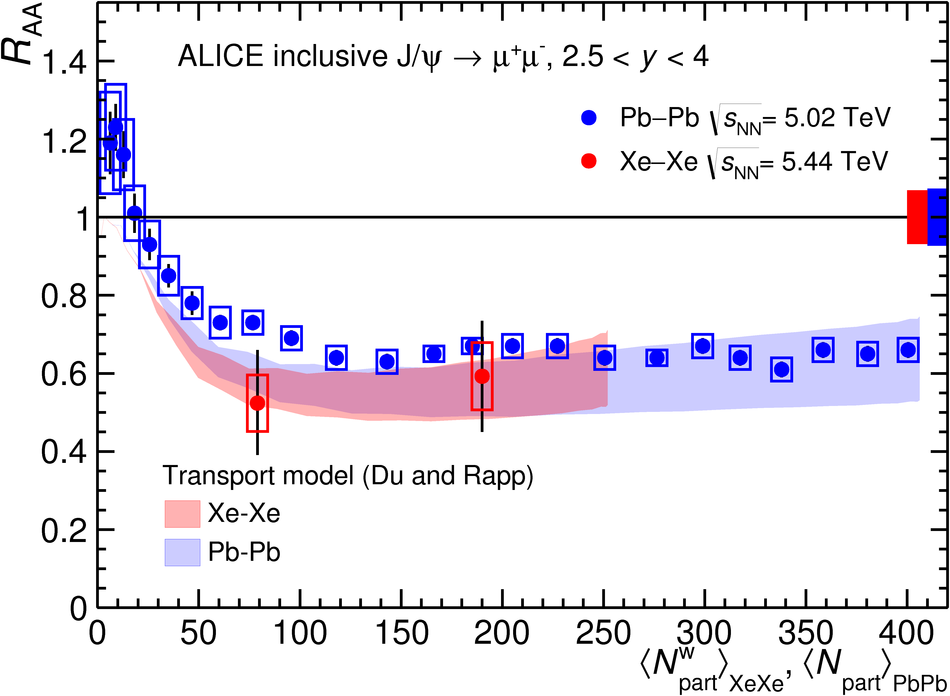Figure 2

 The inclusive J/$\psi$ nuclear modification factor for \mbox{Xe--Xe} collisions at $\sqrt{s_{\rm NN}}=5.44$ TeV. The results are plotted using as centrality variable $\langle N^{\rm w}_{\rm part}\rangle$, obtained by weighting, in each centrality interval, the $N_{\rm part}$ distribution with the corresponding distribution of the number of nucleon-nucleon collisions. The error bars represent the statistical uncertainties, the boxes around the points the uncorrelated systematic uncertainties. Correlated uncertainties are shown as a filled box around unity The results are compared with the same quantity for \mbox{Pb--Pb} collisions at $\sqrt{s_{\rm NN}}=5.02$ TeV~ and to the results of the calculation of a transport model~. For \mbox{Pb--Pb}, the weighting of $N_{\rm part}$ with the number of nucleon-nucleon collisions was not performed, since it leads to a negligible effect when the centrality intervals are narrow.